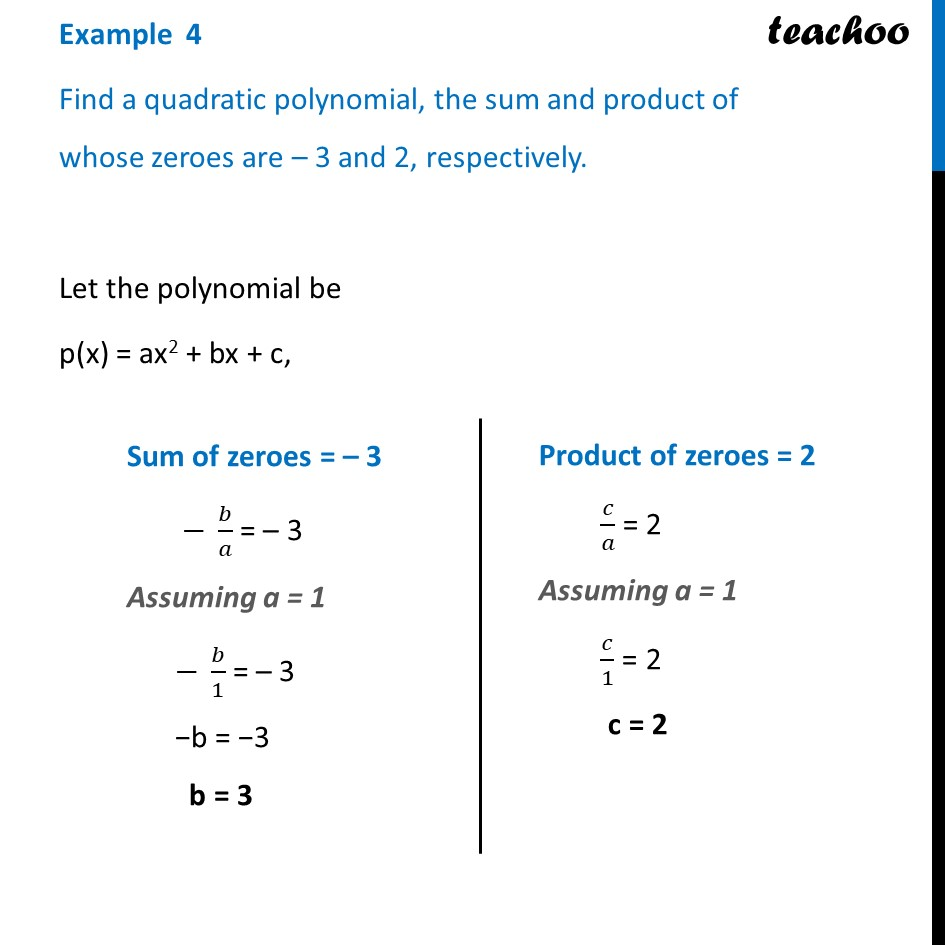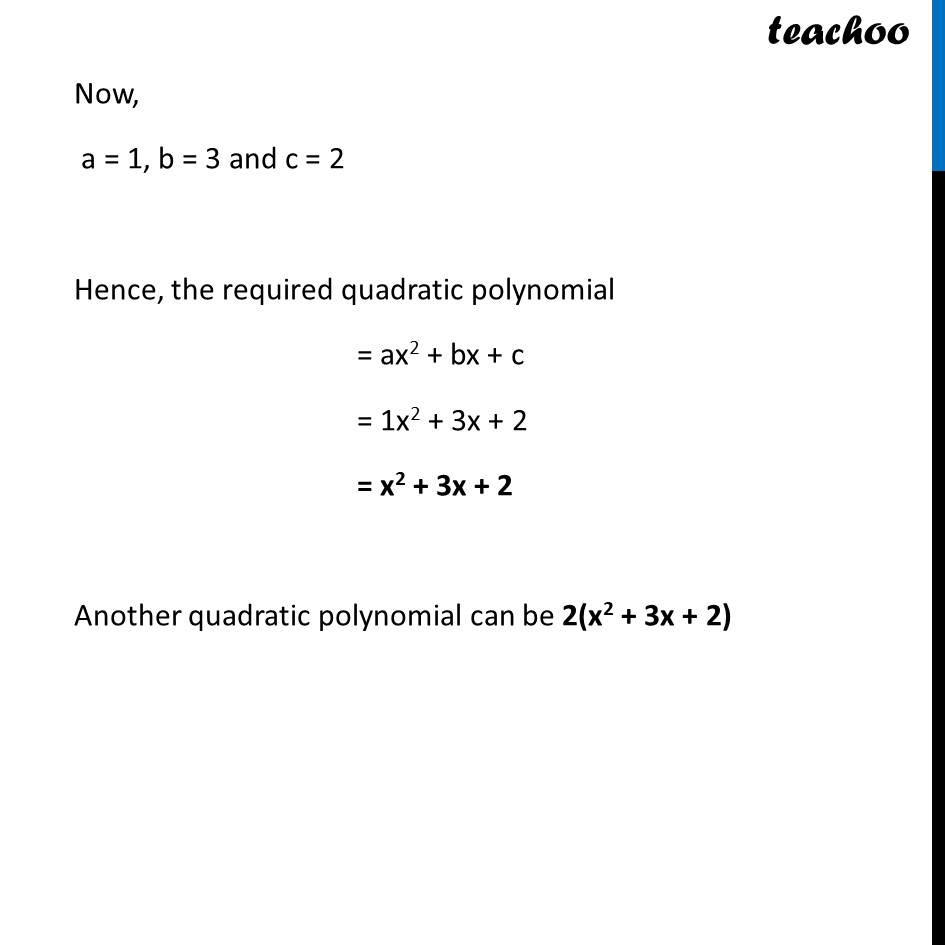Chapter 2 Class 10 Polynomials

Class 10
Important Questions for Exam - Class 10Learn in your speed, with individual attention - Teachoo Maths 1-on-1 Class

### Transcript

Example 4 Find a quadratic polynomial, the sum and product of whose zeroes are – 3 and 2, respectively. Let the polynomial be p(x) = ax2 + bx + c, Sum of zeroes = – 3 − 𝑏/𝑎 = – 3 Assuming a = 1 − 𝑏/1 = – 3 −b = −3 b = 3 Product of zeroes = 2 𝑐/𝑎 = 2 Assuming a = 1 𝑐/1 = 2 c = 2